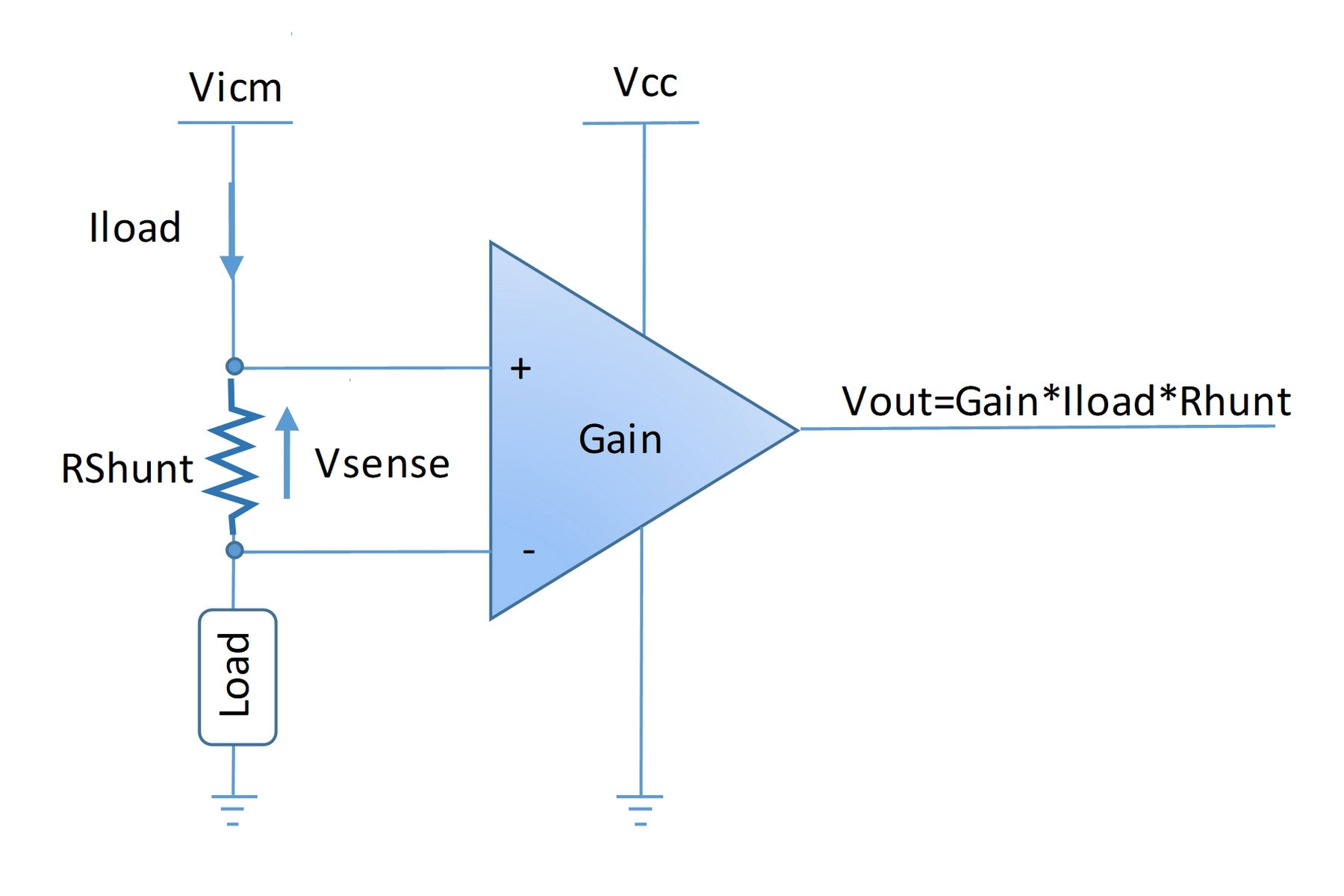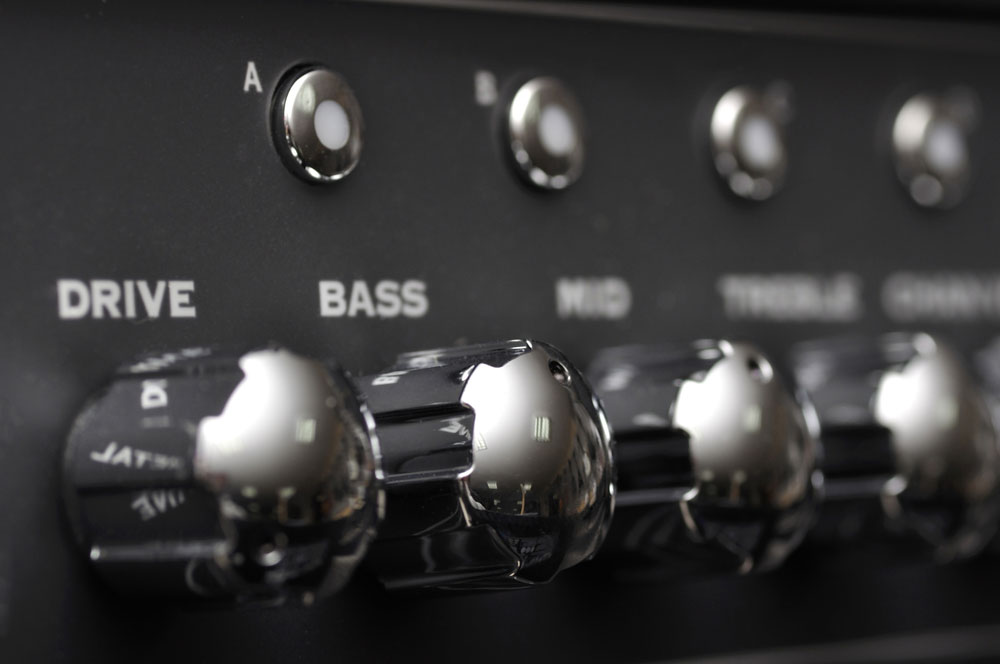Blog  /  Current Sense Amps: Capture Small Voltage Drop With Precision

# Current Sense Amps: Capture Small Voltage Drop With Precision

If you want to track the energy flow in your circuit system, you will need current sensing solutions. The solution might be an amplifier, but it is vital to ensure the power settings are efficient. Moreover, you can avoid overheating, short circuits, and overcurrent protection. While using a current sense amps, you must consider high voltage systems. A sensing amp may help handle the negative voltages and secure the high power system. Similarly, you will see how precise the device is in capturing small voltage drops. In this way, it will allow less energy dissipation across the circuit.

## What is a Current Sense Amp?

We define a current sense amp as a differential amplifier that provides a voltage as output, proportional to the current load value. Here, the current load value is current, passing through the load side of the amplifier. Thus, the greater is the flow of current, the higher is the voltage produced in the system.mother board of computer

The current sensing amplifiers may provide various benefits to the system engineers as they can:

• Measure a wide range of current, including few milliamperes to hundreds
• Check real-time current measurement in the circuit topology
• Test the current with high precision
• Keep the input common-mode voltage in the normal range, much greater than the power supply rails
• Shrink the Printed Circuit Board (PCB) size

## Working of a Current Sense AmplifierCurrent Sense Amplifier Circuit Diagram

Source:https://www.st.com/en/amplifiers-and-comparators/current-sensing.html

A current sense amp works on the principle of Ohm’s Law. It states that the voltage in a circuit is directly proportional to the current passing. Hence, passing the current through a shunt resistor Rs results in the voltage drop (Vsense). Usually, this released sense voltage is much less. So that you can control the dissipation of power and the energy loss.

Next, the drop voltage is amplified with the help of the internal instrumentation amplifier. Thus, you get your desired output voltage Vout. For refining the signal more, you can process the Vout with an Analog to Digital converter or ADC.

## Difference between Common Amplifier and Current Sense Amp

As the name suggests, common amplifiers and current sensing amplifiers belong to the same class. Yet they are designed for different purposes. At this point, it is important to define two parameters, which are essential for both types of op-amps.

• Common Mode Voltage: It is the average voltage applied on the input points of an amp. The input offset voltage is essential, as every amplifier has its tolerance level.
• CMRR: It is the ratio between common-mode gain and differential gain in an amplifier. The common-mode rejection ratio shows how many common-mode signals a device will reject. In practice, you can amplify the signals without high noise interference, using high CMRR.

Standard amplifiers are not able to amplify small voltage signals and have lower CMRR. For operating regular amps, you have to connect the power source between the Vcc and Vee power supply rails. In fact, these amplifiers work on the common ground path signals. Additionally, suppose you try to apply an external voltage to the input pins. In that case, it can cause maximum current to flow across the system and heavy damage.

However, precision current amplifiers can detect and amplify small voltage signals. Also, their CMRR is higher than any other operational amplifiers. Current sense amps can tolerate higher pin voltages than the input voltage. Since the current sensing amplifiers' path control, they can alternate their supply voltage sources in lower Vcc cases.

## Types of Current Sense AmplifiersMusic amplifier control panel

Here are some common types of current sense amps

• High Side Amplifiers; where you will test the current between power supply rail and load. In this case, the power supply will be much lower than the DC voltage on input.
• Low Side Amplifiers, where you will test the current between load and the ground. In this case, the power supply will be closer to the ground value.
• Bidirectional Amplifiers, where the amplifier has one additional Vref pin to set the output reference within the adequate current range.
• Isolated Amplifiers

## Application of Current Sense Amplifiers

### High Side Current Sense Circuits

When you use a high-side current sensing technique, you will mount a high-side shunt resistor between the positive side of your power supply and the active load. As you know, shunt resistors help in measuring the drop voltage across its two ends. Here, you can use it for the same purpose.Source: https://www.apogeeweb.net

Advantages of using the high current sense technique are;

• As you place the shunt resistor between load and ground, you can easily detect the short circuit in this area.
• Also, while getting the measurements, you will be able to capture the exact load current with little to no effort.
• Load is in a proper connection with the ground and has minor disturbance

But, one disadvantage you will face is that you will require devices of high CMRR. It is because the voltage across the resistor is less than the load voltage current.

### Current Sense Amps--Low Side Current Sense Circuits

To implement the low-side current circuits, you will place the shunt current sense resistor between the ground and the active load. In this way, whenever the current flows towards the ground, it will always go through the shunt resistor.Source: https://components101.com

The benefits of using low circuit current technique are

- You can quickly implement it as the sense voltage is referenced to the ground

- Small voltage current is produced across the resistor with less common-mode voltage error

- Also, a low CMRR device can work very well

- Overall, the solution is cost-effective

Yet, the arrangement can result in offset load from the ground. Hence, it is unable to detect a high flow of current, and thus, a short circuit happens.

### Current Sense Amps--Bidirectional Current Sense Circuits

The bidirectional current sense technique is complex than the former two methods. In this technique, you have to sense the current in both ways. For that, you will need amplifiers that can detect the current flow in both directions.Source: https://components101.com

In this technique, the only thing you will require is reverse polarity protection and a suitable switching mechanism to change the pathways as the polarity of the source changes.

Another way to detect the bidirectional current is to compare the voltage drop value with the input voltage current. If the voltage drop is more significant than the input, the current is in one direction. Otherwise, the current waves are opposite to each other.

### Current Sense Amps--Isolated Current Sense Circuits

The use of isolated current sensing requires hall effect induction or a proper transformer. The induction will create voltage on the side of the transformer and the current flow on the other side. The current and voltage will be proportional to each other.Source: https://www.apogeeweb.net/electron/what-is-a-current-sense-amplifier-overview.html

## Design Considerations for using Current Sense Amplifier

For creating a current sense circuit where the line is 12 V and 1A, you will need a current sense amplifier. However, you have to choose the one, which can work with a 12V supply. Also, it should be able to sense current more than 1A.

Note that as there is no bidirectional current, in this case, you will choose between the high or low current sensing amplifier. The high current amp will help you detect the short circuit or any error sources.Source: https://components101.com/articles/basics-of-current-sense-amplifier-its-types-and-design-guide

Here, as the load is an inductor or capacitor, there is a chance that an inrush current will arise. Hence, you will choose a resistor with less resistance. For instance, in the above circuit, LT6108 is used as the voltage range for this amplifier is 2.7V-60V. Moreover, the common-mode rejection ratio is up to 125dB, which is a higher value.

## Conclusion

The current sense amps use the current shunt motor to amplify the output voltage, proportional to the input current. To cater to various electrical problems, there is a wide variety of amplifiers from which you can choose the suitable one. However, every category comes with its challenges and advantages.

Here at OurPCB, we offer PCB assembly with attention to detail. To avail of our services, contact us now.